# Decimal fraction

(diff) ← Older revision | Latest revision (diff) | Newer revision → (diff)

An arithmetical fraction with an integral power of 10 as its denominator. The following notation has been accepted for a decimal fraction: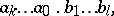(1)

whereare integers and if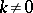then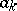is also non-zero.

Formula (1) expresses the number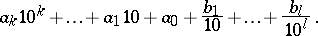For example,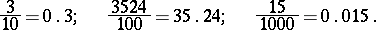The digits to the right of the decimal point are known as the decimal digits. If a decimal fraction contains no integer part, i.e. its absolute value is smaller than one, a zero is placed to the left of the decimal point.

An infinite decimal fraction is an infinite sequence of digits such as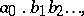(2)

whereis an integer, while each one of the numbers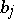,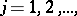assumes one of the values. Any real numberis the sum of such a series, i.e.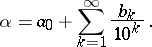The partial sums of the series (2) are finite decimal fractions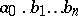, which are approximate values of the numbersmaller than; the numbers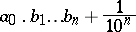are the respective approximate values larger than. If there exists integersandsuch that for allthe equalitiesare valid, the infinite decimal fraction is said to be periodic. Any finite decimal fraction may be regarded as an infinite periodic fraction with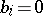for. Ifis a rational number, the corresponding fraction (2) will be periodic. Ifis irrational, the fraction (2) cannot be periodic.

How to Cite This Entry:
Decimal fraction. S.A. Stepanov (originator), Encyclopedia of Mathematics. URL: http://www.encyclopediaofmath.org/index.php?title=Decimal_fraction&oldid=16491
This text originally appeared in Encyclopedia of Mathematics - ISBN 1402006098